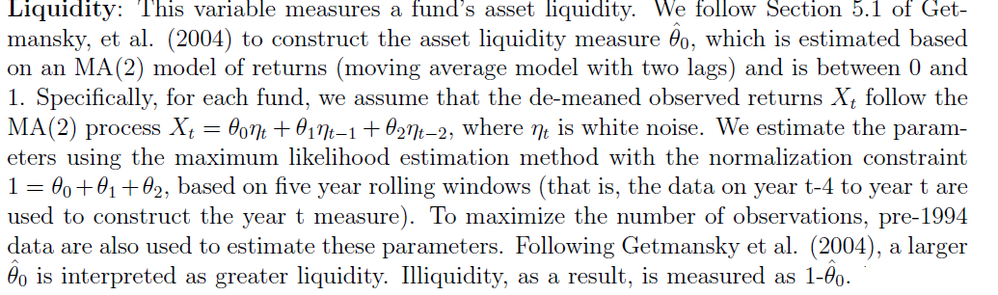## MA(2)

Hi,

I am hoping this would be my last question,

I am trying to measure a fund’s asset liquidity, which is estimated based
on an MA(2) model of returns (moving average model with two lags) and is between 0 and1.

Date       Return     AUM    mainstrategy

199512   -0.0055   26.9     Relative value

199601    0.0048   27.1      Relative value

199602    0.0089   30.7      CTA6 REPLIES 6Ksharp
Super User

## Re: MA(2)

Suggest you post it at here

Forecasting and Econometrics

https://communities.sas.com/t5/Forecasting-and-Econometrics/bd-p/forecasting_econometrics

Since it is a question related to SAS/ETS

## Re: MA(2)

What tools do you want to use to solve this problem? You posted to the SAS/IML discussion forum, but your question is not directly related to matrix programming.

## Re: MA(2)

Hi Rick,

I posted to the wrong forum. I posted it again to the appropriate forum. However I will be glad if you could help me.

proc arima data = sample ;
by mainstrategy;

identify var=returns nlag=6 outcov=acf noprint ;

estimate q=2;

run ;

Is there a problem with using the by statement in arima procedure. Is there another way to achieve the same results without using the by statement. I am applying a second order moving average process (MA(2)) to uncover the
unobserved returns.

𝑅𝑡0 = 𝜃0𝑅𝑡 + 𝜃1𝑅𝑡−1 + 𝜃2𝑅𝑡−2, (1)
with 𝜃𝑗 ∈ [0,1], 𝑗 = 0,1,2, (2)
and 1 = 𝜃0 + 𝜃1 + 𝜃2.

I am trying to estimate the parameters of the model for each hedge fund strategy by maximum likeli-
hood. Then the estimated parameters will be used to desmooth returns.Ksharp
Super User

## Re: MA(2)

OK. Here is what I got . IML is awesome .

I use OLS to estimate theta0+theta1+theta2=1 . Not ML , I think both could be accepted.

Good Luck.

``````

data have;
call streaminit(1234);
length 	mainstrategy \$ 20;
do mainstrategy='Relative value','CTA';
do year=1990 to 2010;
do month=1 to 12;
date=mdy(month,1,year);
return=rand('normal');
noise=rand('normal');
output;
end;
end;
end;
drop year month ;
format date yymmn6.;
run;

proc iml;
use have nobs nobs;
read all var{mainstrategy date return noise};
close;

start_end=t(loc( mainstrategy^=t( {' '}||remove(mainstrategy,nobs) ) ))||
t(loc( mainstrategy^=t( remove(mainstrategy,1)||{' '}) ) );

theta0=j(nobs,1,.);
theta1=j(nobs,1,.);
theta2=j(nobs,1,.);

do i=1 to nrow(start_end);
do j=start_end[i,1] to start_end[i,2]-2-5*12;

x=return[j:j+5*12]||noise[j:j+5*12]||noise[j+1:j+1+5*12]||noise[j+2:j+2+5*12];

create temp from x[c={y noise0 noise1 noise2}];
append from x;
close;

submit;
proc reg data=temp outest=est noprint;
model y=noise0 noise1 noise2;
restrict noise0+noise1+noise2=1;
run;
endsubmit;

use est;
close;

theta0[j,1]=noise0;
theta1[j,1]=noise1;
theta2[j,1]=noise2;

end;
end;

create MA2 var{mainstrategy date return noise theta0 theta1 theta2};
append;
close;
quit;``````

## Re: MA(2)

HI XIa,
Thanks for the rely but i was wondering is it appropriate to use the ml method? since i am estimating the parameters by maximum likelihood?Ksharp
Super User

## Re: MA(2)

No. I don't know how to use ML(proc genmod) to estimate theta0+theta1+theta2=1 .

From The DO Loop